free newsletter!tell a friend!contestssoftware
reviews
bestseller list
price survey
what's new
product support
searcheducational tools
math worksheets
vocabulary builders
hangman
logic games
brain foodeducational insightsfeature articlesmarketplace
movie cornerSuperKids home
humor
help* * *

Promotions

* * *math worksheets > > fractions > > multiplying fractions

## SuperKids Math Review

### How to Multiply Fractions

 Remember . . .Here's a memory trick: the Denominator is the bottom, or Down number in a fraction -- and both Denominator and Down start with the letter D.

Multiplying fractions is simple. Unlike adding and subtracting, where care must be taken to ensure that both fractions have a common denominator, the multiplication operation has no such requirement.

Method 1 - (for beginners) Just multiply the numerators (top numbers), and the denomominators (bottom numbers), and place the resulting answers in their respective top / bottom location in the answer fraction. Then reduce the fraction, if possible

Example 1: Simple fraction multiplicationx=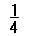No reduction is possible, so we have found the answer!

Example 2: Multiplication requiring answer fraction to be reducedx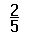=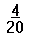Then reduce:=Example 3: Multiplication with a mixed numberx= ?

First convert the mixed number to an improper fraction:=Then multiply:x=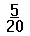Then reduce the fraction:=Method 2 - (for anyone who understands the above concept). Before multiplying the numerators and denominators, look for ways to pre-reduce the fractions, both within each fraction, and across the fractions.

Example 1: Pre-reducing within a fractionx= ?

First reduce the 2/4 to 1/2, then multiply the numerators (top numbers), and the denominators (bottom numbers), and place the resulting answers in their respective top / bottom location in the answer fraction. Then reduce the fraction, if possible.x=x=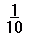Example 2: Reducing across fractionsx= ?

Here we divide the first numerator (5) by the second denominator (5), before multiplying the numerators (top numbers), and the denomominators (bottom numbers).x=x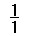=This method has the advantage of allowing the use of smaller numbers in the calculation process.

Got it? Great! Then go to the SuperKids Math Worksheet Creator for Basic Fractions, and give it a try!Questions or comments regarding this site? webmaster@superkids.com